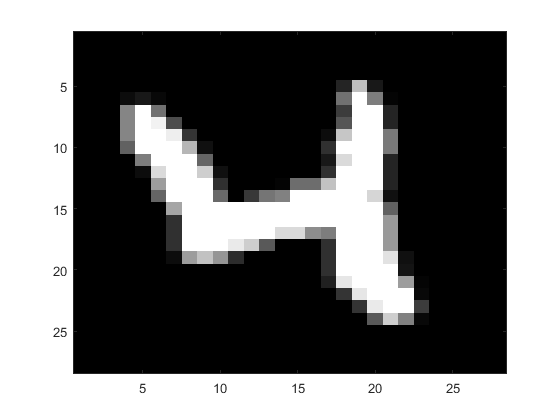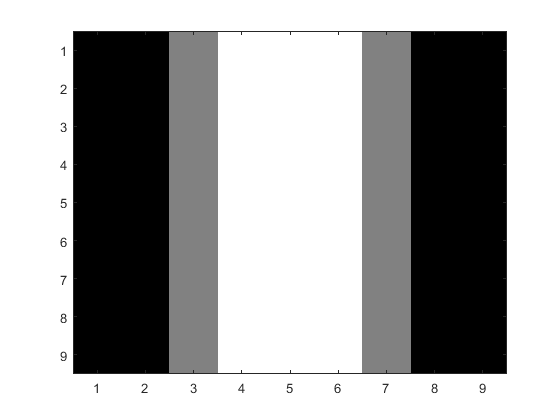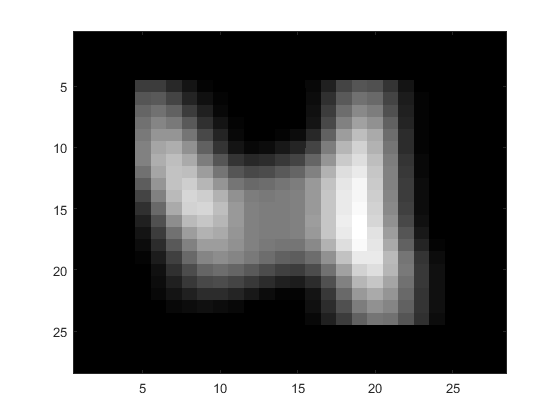# Problem 58877. Neural Net Image Convolution: Return only Valid portion of conv2

This challenge is to return only the Valid portion of a 2-D convolution. Valid is deemed to be where all elements of the kernel are applied against the image. The (1,1) point with a ones(3) kernel would be invalid while point (2,2) would return a Valid convolution value. The images will be even-square,m, and the kernels will be odd-square,K.The m=ones(6) and K=ones(3) would return a 4x4 matrix of 9s.
mValidConv2=create_ValidConv2(m,K)
In neural net processing of the Mnist database of digits(0-9) one Convolutional Neural Network method uses 9x9 kernels on the 28x28 images to return the Valid 20x20 central core for the next processing step. The third image includes a black border of four around Valid to maintain scale.The entire Matlab code from Convolutional Neural Networks in Matlab by Nuruzzaman Faruqui , a Matlab Neural Net Tutorial for Mnist processing, is included in the function template. Loading the Mnist Train/Test Images/Labels from posted MAT files or THE MNIST DATABASE of handwritten digits are provided. The Neural Net tutorial explains Neural Net processing for Digit Recognition. The neural net training method uses 784 input nodes, 20 convolutions(9x9), ReLU, Pooling, batch sampling, single hidden layer of 100 nodes, updating parameters via backwards propagation, and updating convolution kernels. The digit prediction accuracy is >94% after 50s of training. The final feature kernels are very amorphous and unexpected.

### Solution Stats

60.0% Correct | 40.0% Incorrect
Last Solution submitted on Sep 24, 2023

### Community Treasure Hunt

Find the treasures in MATLAB Central and discover how the community can help you!

Start Hunting!